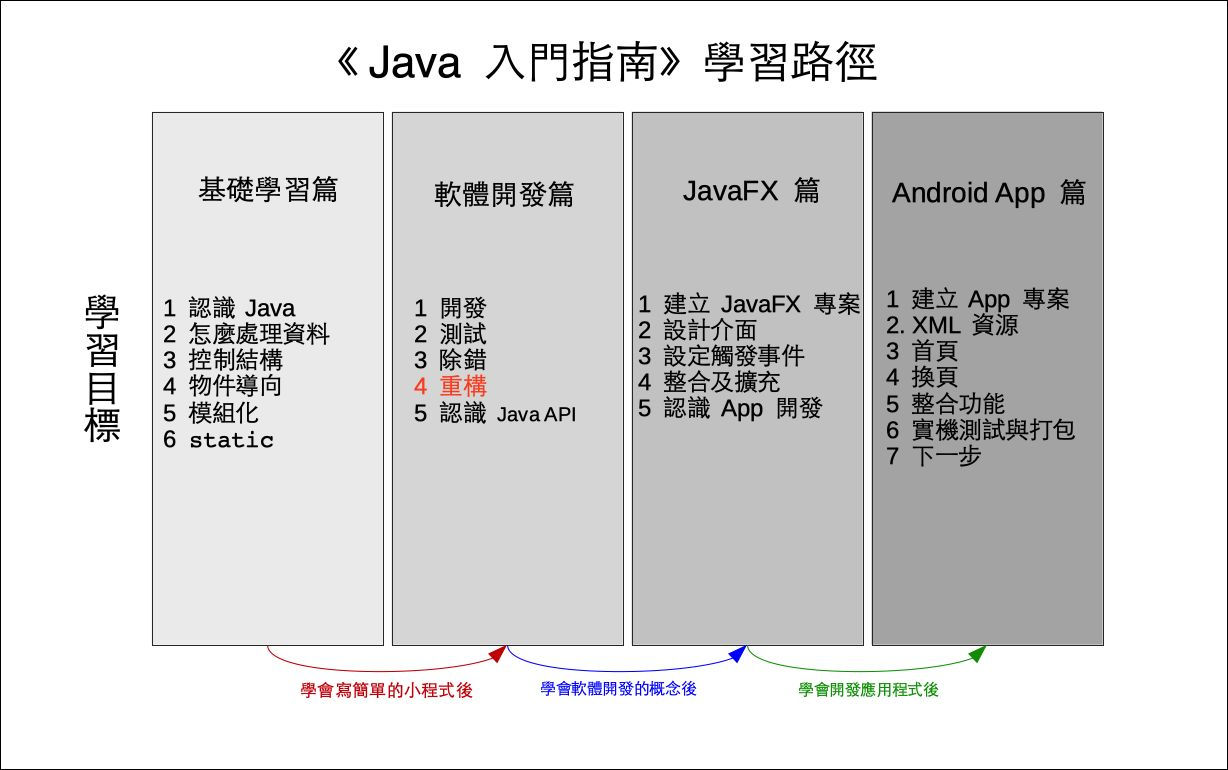# 單元 18 - 重構

～～學習進度表～～Refactoring

5 × 10 = 50

1 × 2 × 3 × ⋯⋯ × 25 × 26 = 4.0329 × 1026

``````import java.util.ArrayList;
import java.util.Arrays;
import java.util.List;
import java.util.Collections;``````

``````// 字母表
final String letter = "abcdefghijklmnopqrstuvwxyz";
// 密碼表
String code;``````

``````// 無參數建構子
public Encrypt() {
this.code = "";
List<String> letterList = new ArrayList<String>(Arrays.asList(this.letter.split("")));
Collections.shuffle(letterList);
for (String s : letterList) {
this.code += s;
}

System.out.println(this.code);
}``````

``this.code = "";``

``List<String> letterList = new ArrayList<String>(Arrays.asList(this.letter.split("")));``

``Collections.shuffle(letterList);``

``````for (String s : letterList) {
this.code += s;
}``````

``System.out.println(this.code);``

``````// 有參數的建構子
public Encrypt(String str) {
this.code = str;
}``````

``````// 編碼方法
public String toDecode(String inputString) {
String result = "";
List<String> inputList = new ArrayList<String>(Arrays.asList(inputString.split("")));
for (String s : inputList) {
if (letter.contains(s)) {
result += this.code.substring(this.letter.indexOf(s), this.letter.indexOf(s)+1);
}
else {
result += s;
}
}

return result;
}``````

``String result = "";``

``List<String> inputList = new ArrayList<String>(Arrays.asList(inputString.split("")));``

``````for (String s : inputList) {
if (letter.contains(s)) {
result += this.code.substring(this.letter.indexOf(s), this.letter.indexOf(s)+1);
}
else {
result += s;
}
}``````

``return result;``

``````// 解碼方法
public String toDecode(String inputString) {
String result = "";
List<String> inputList = new ArrayList>String>(Arrays.asList(inputString.split(""s)));
for (String s : inputList) {
if (letter.contains(s)) {
result += this.letter.substring(this.code.indexOf(s), this.code.indexOf(s)+1);
}
else {
result += s;
}
}

return result;
}``````

 rkuodvxlmyasicgehtnqjpbzwf There is no spoon. Tldtd mn cg neggc. There is no spoon.algorithm演算法
array陣列
class類別
constructor建構子
field屬性
identifier識別字
keyword關鍵字
local variable區域變數
loop迴圈
method方法
parameter參數
refactoring重構
software軟體
source code原始碼
string字串

1. The Java™ Tutorials: Declaring Classes
2. The Java™ Tutorials: Declaring Member Variables
3. The Java™ Tutorials: Defining Methods
4. The Java™ Tutorials: Providing Constructors for Your Classes
5. The Java™ Tutorials: Returning a Value from a Method
6. The Java™ Tutorials: Using the this Keyword